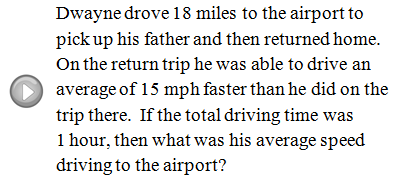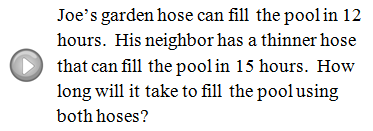Showing posts with label Distance. Show all posts
Showing posts with label Distance. Show all posts

Wednesday, June 26, 2013

Distance and Midpoint Formulas

In this section we will review the distance and midpoint formulas.
Notice that the distance formula results in a real number and that the midpoint formula results in an ordered pair.

[ Interactive: Distance and Midpoint ]

Given two points find the distance and midpoint between them.

Video Lessons:

Use the distance and/or midpoint formulas to solve the following.

Example: If the diameter of a circle is defined by the two points (-3, 4) and (7, 4), find the center and radius of the circle. (Hint: diameter = 2r)
Example: Find the area of a circle given center (-3, 3) and point (3, 3) on the circle.

YouTube Example: Find the coordinates of an endpoint given the other endpoint and the midpoint.
---

Monday, April 22, 2013

Interactive: Distance and Midpoint

Interactive Instructions: Move the green points A and B. Hover over the points to see the coordinates. You can also drag the line segment.

Tuesday, November 6, 2012

Applications of Rational Equations

The set-up for the applications in this chapter will include rational equations. The keyword, reciprocal, appears often. Remember that the reciprocal of a number is 1 divided by that number.

Number Problems
Example: One positive integer is 5 more than the other.  When the reciprocal of the larger number is subtracted from the reciprocal of the smaller the result is 5/14. Find the two integers.
Example: The difference between the reciprocals of two consecutive positive odd integers is 2/15. Find the integers.

Work-Rate Problems
There are two equivalent formulas to choose from when solving work-rate problems. If two people are working together on a job then their work rates add and they can perform the job working together in a shorter amount of time. If we let x = the time it takes a person to complete a task then his work rate is 1/x. In other words, he can complete the 1 job in x number of hours. If we let y = the time it takes a second person to complete the task and t = the time it takes for both people working together we get the following work-rate formulas:
Example: Bill’s garden hose can fill the pool in 12 hours.  His neighbor has a hose that can fill the pool in 15 hours.  How long will it take to fill the pool using both hoses?
Example: Joe can complete his yard work in 3 hours by himself.  If is son helps it will only take 2 hours working together. How long would the yard work take if is son was working alone?
Example: Norm and Cliff can paint the office in 5 hours working together. Being a professional painter, Norm can paint twice as fast as Cliff. How long would it take Cliff to paint the office by himself?
Work-Rate Problem: One small water hose takes twice as long to fill the basin as the larger water hose. If the two hoses working together take 40 minutes to fill the basin how long would it have taken each hose to individually fill the basin?
Work-Rate Problem: Joe can texture and paint a room in 8 hours working alone. It would take Bill 6 hours to do the same job. How long will it take them to complete the job working together?
Work-Rate Problem: Two printers, working at the same rate, work together printing payroll checks for 4 hours before one breaks down. The other takes 3 more hours to complete the job working alone. How long would it have taken only one printer to complete the job?
Uniform Motion Problems (Distance Problems)
We have set up uniform motion problems using the formula D = r * t. For the following motion problems, we will need the equivalent formula D/r = t to set up the equations.

Example: The first leg of Mary’s road trip consisted of 120 miles of traffic.  When the traffic cleared, she was able to drive twice as fast for 300 miles. If the total trip took 9 hours, then for how much time was she stuck in traffic?
Example: A passenger train can travel 20 miles per hour faster than a freight train.  If the passenger train can cover 390 miles in the same time it takes the freight train to cover 270 miles, how fast is each train?
Example: Billy rode his skateboard 24 miles to his grandmother’s house for the day. It was a rough ride so he borrowed his grandmother’s bicycle for the return trip. Going twice as fast on the bicycle the return trip took 2 hours less time.  What was his average speed on the bicycle?

Example: Brett lives on the river 45 miles upstream from town.  When the current is 2 miles per hour he can row his boat downstream to town for supplies and back in 14 hours.  What is his average rowing speed in still water?